Engineering ToolBox - Resources, Tools and Basic Information for Engineering and Design of Technical Applications!

# Shafts Torsion

## The torsion of solid or hollow shafts - Polar Moment of Inertia of Area.

### Shear Stress in the Shaft

When a shaft is subjected to a torque or twisting a shearing stress is produced in the shaft. The shear stress varies from zero in the axis to a maximum at the outside surface of the shaft.

The shear stress in a solid circular shaft in a given position can be expressed as:

τ = T r / J                              (1)

where

τ = shear stress (Pa, lbf/ft2 (psf))

T = twisting moment (Nm, lbf ft)

r = distance from center to stressed surface in the given position (m, ft)

J = Polar Moment of Inertia of Area (m4, ft4)

Note

• the "Polar Moment of Inertia of an Area" is a measure of a shaft's ability to resist torsion. The "Polar Moment of Inertia" is defined with respect to an axis perpendicular to the area considered. It is analogous to the "Area Moment of Inertia" - which characterizes a beam's ability to resist bending - required to predict deflection and stress in a beam.
• 1 ft = 12 in
• 1 ft4 = 20736 in4
• 1 psf (lbf/ft2) = 1/144 psi (lbf/in2)

"Polar Moment of Inertia of an Area" is also called "Polar Moment of Inertia", "Second Moment of Area", "Area Moment of Inertia", "Polar Moment of Area" or "Second Area Moment".

#### Polar Moment of Inertia vs. Area Moment of Inertia

• "Polar Moment of Inertia" - a measure of a beam's ability to resist torsion - which is required to calculate the twist of a beam subjected to torque
• "Area Moment of Inertia" - a property of shape that is used to predict deflection, bending and stress in beams

### Circular Shaft and Maximum Moment or Torque

Maximum moment in a circular shaft can be expressed as:

Tmax = τmax J / R                               (2)

where

Tmax = maximum twisting torque (Nm, lbf ft)

τmax = maximum shear stress (Pa, lbf/ft2)

R = radius of shaft (m, ft)

Combining (2) and (3) for a solid shaft

Tmax = (π / 16) τmax D3                            (2b)

Combining (2) and (3b) for a hollow shaft

Tmax = (π / 16) τmax (D4 - d4) / D                             (2c)

### Circular Shaft and Polar Moment of Inertia

Polar Moment of Inertia of a circular solid shaft can be expressed as

J = π R4 / 2

= π (D / 2)4 / 2

= π D4 / 32                          (3)

where

D = shaft outside diameter (m, in)

Polar Moment of Inertia of a circular hollow shaft can be expressed as

J = π (D4 - d4) / 32                          (3b)

where

d = shaft inside diameter (m, ft)

### Diameter of a Solid Shaft

Diameter of a solid shaft can calculated by the formula

D = 1.72 (Tmax / τmax)1/3                            (4)

### Torsional Deflection of Shaft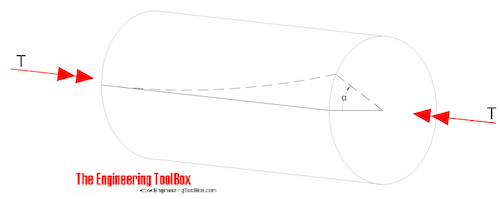The angular deflection of a torsion shaft can be expressed as

α = L T / (J G)                                  (5)

where

α = angular shaft deflection (radians)

L = length of shaft (m, ft)

G = Shear Modulus of Rigidity - or Modulus of Rigidity (Pa, psf)

The angular deflection of a torsion solid shaft can be expressed as

α = 32 L T / (G π D4)                             (5a)

The angular deflection of a torsion hollow shaft can be expressed as

α = 32 L T / (G π (D4- d4))                              (5b)

The angle in degrees can be achieved by multiplying the angle θ in radians with 180 / π.

Solid shaft (π replaced)

αdegrees ≈ 584 L T / (G D4)                              (6a)

Hollow shaft (π replaced)

αdegrees ≈ 584 L T / (G (D4- d4)                            (6b)

### Torsion Resisting Moments from Shafts of Various Cross Sections

Shafts - Torsional Resisting Moments
Shaft Cross Section AreaMaximum Torsional
Resisting Moment
- Tmax -
(Nm, lbf ft)
Nomenclature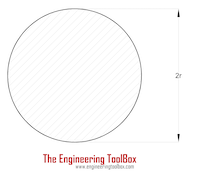Solid Cylinder Shaft

(π / 16) τmax (2 r)3

= (π / 16) τmax D3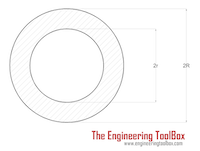Hollow Cylinder Shaft

(π / 16) τmax ((2 R)4 - (2 r)4) / (2 R)

= (π / 16) τmax (D4 - d4) / D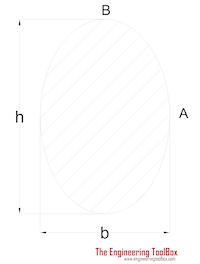Ellipse Shaft (π / 16) τmax b2 h h = "height" of shaft
b = "width" of shaft
h > b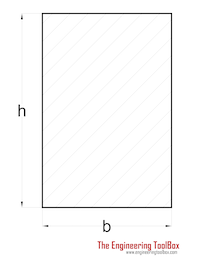Rectangle Shaft (2 / 9) τmax b2 h h > b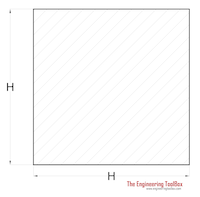Square Shaft (2 / 9) τmax H3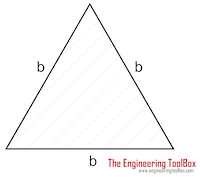Triangle Shaft (1 / 20) τmax b3 b = length of triangle side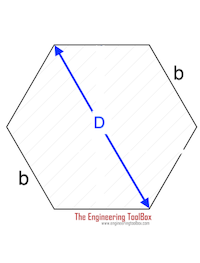Hexagon Shaft

0.123 τmax D3

0.189 τmax b3

### Example - Shear Stress and Angular Deflection in a Solid Cylinder

A moment of 1000 Nm is acting on a solid cylinder shaft with diameter 50 mm (0.05 m) and length 1 m. The shaft is made in steel with modulus of rigidity 79 GPa (79 109 Pa)

Maximum shear stress can be calculated as

τmax = T r / J

= T (D / 2) / (π D4 / 32)

= (1000 Nm) ((0.05 m) / 2) / (π (0.05 m)4 / 32)

= 40764331 Pa

= 40.8 MPa

The angular deflection of the shaft can be calculated as

θ = L T / (J G)

= L T / ((π D4 / 32) G)

= (1 m) (1000 Nm) / ((π (0.05 m)4 / 32) (79 109 Pa))

= 1.2 o

### Example - Shear Stress and Angular Deflection in a Hollow Cylinder

A moment of 1000 Nm is acting on a hollow cylinder shaft with outer diameter 50 mm (0.05 m), inner diameter 30 mm (0.03 m) and length 1 m. The shaft is made in steel with modulus of rigidity 79 GPa (79 109 Pa)

Maximum shear stress can be calculated as

τmax = T r / J

= T (D / 2) / (π (D4 - d4) / 32)

= (1000 Nm) ((0.05 m) / 2) / (π ((0.05 m)4 - (0.03 m)4) / 32)

= 46.8 MPa

The angular deflection of the shaft can be calculated as

θ = L T / (J G)

= L T / ((π D4 / 32) G)

= (1 m) (1000 Nm) / ((π ((0.05 m)4 - (0.03 m)4) / 32) (79 109 Pa))

= 1.4 o

### Example - Required Shaft Diameter to Transmit Power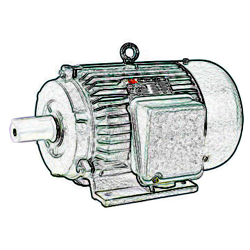A 15 kW electric motor shall be used to transmit power through a connected solid shaft. The motor and the shaft rotates with 2000 rpm. The maximum allowable shear stress  - τmax - in the shaft is 100 MPa.

The connection between power and torque can be expressed

P = 0.105 nrpm T                    (7)

where

P = power (W)

nrpm = speed of shaft (rpm)

Re-arranged and with values - the torque can be calculated

T = (15 103 W) / (0.105 (2000 rpm))

= 71 Nm

Minimum diameter of the shaft can be calculated with eq. 4

D = 1.72 ((71 Nm) / (100 106 Pa))1/3

= 0.0153 m

= 15.3 mm

## Related Topics

• ### Mechanics

Forces, acceleration, displacement, vectors, motion, momentum, energy of objects and more.
• ### Statics

Loads - forces and torque, beams and columns.

## Related Documents

• ### Improvised Torque Wrench

Improvise a torque wrench with a luggage scale.
• ### Mass Moment of Inertia

The Mass Moment of Inertia vs. mass of object, it's shape and relative point of rotation - the Radius of Gyration.
• ### Mild Steel - Round Bars Weight

Weight of round bars.
• ### Modulus of Rigidity

Shear Modulus (Modulus of Rigidity) is the elasticity coefficient for shearing or torsion force.
• ### Rotating Shafts - Torque

Torsional moments acting on rotating shafts.
• ### Stiffness

Stiffness is resistance to deflection.
• ### Stress

Stress is force applied on cross-sectional area.
• ### Stress in Thick-Walled Cylinders or Tubes

Radial and tangential stress in thick-walled cylinders or tubes with closed ends - with internal and external pressure.
• ### Torque - Work done and Power Transmitted

The work done and power transmitted by a constant torque.

## Engineering ToolBox - SketchUp Extension - Online 3D modeling!

Add standard and customized parametric components - like flange beams, lumbers, piping, stairs and more - to your Sketchup model with the Engineering ToolBox - SketchUp Extension - enabled for use with older versions of the amazing SketchUp Make and the newer "up to date" SketchUp Pro . Add the Engineering ToolBox extension to your SketchUp Make/Pro from the Extension Warehouse !

We don't collect information from our users. More about

## Citation

• The Engineering ToolBox (2005). Shafts Torsion. [online] Available at: https://www.engineeringtoolbox.com/torsion-shafts-d_947.html [Accessed Day Month Year].

Modify the access date according your visit.

9.27.11